## Welcome to the World of Modelling and Simulation

### What is Modelling?

This blog is all about system dynamics modelling and simulation applied in the engineering field, especially mechanical, electrical, and ...### Discussion on Actuator Saturation in Control System

This lab concerns a special non-linear effect in control system which happens when the control signal becomes larger than the actuator limit. In this situation, the feedback path is broken and the actuator runs independently. The control signal increases which further creates large transients in the system to get the error down to negative. If there is an integrator in the controller, then this would keep integrating the error to produce large control signal above the actuator saturation limit. So, the objectives of this lab are to study the effects of integrator “Windup” phenomenon and design an anti windup compensator to avoid this condition. The lab report is organized in three steps according to the lab manual instruction.

## According to the lab procedure, the analog output is connected to the analog input by RCS cable on the data acquisition board. Then a SIMULINK model is developed for setting saturation limit which is shown in figure 1. A PID controller has been introduced where the values of Kp, Ki and Kd are set to 1, 1 and 0 respectively. For real time simulation, HIL read and write analog blocks are connected to the model. For these blocks, the analog input-output voltage is restricted to ±10 volt. Therefore, in the following SIMULINK model, the input signal limit is set to ±10 volt and output signal limit is set to ±5 volt. The input sine wave has amplitude of 15 Volt and frequency of 1 rad/sec. The input sine wave, saturated input signal, signal from the integrator and the output signal are highlighted in figure 2. For the simulation, the solver is chosen ODE1 (Euler) with fixed step size 0.002. This solver is used for each and every simulation in this work.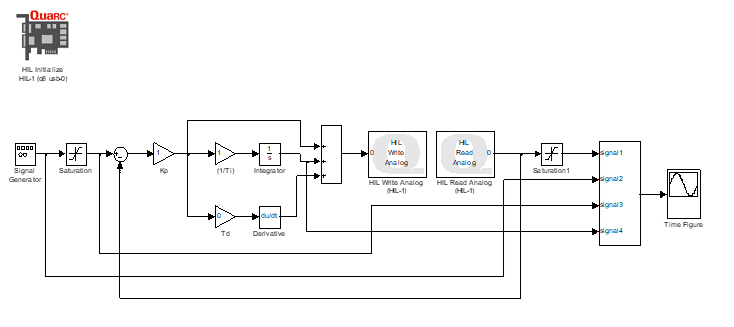Figure 1: SIMULINK model for experimenting saturation limit.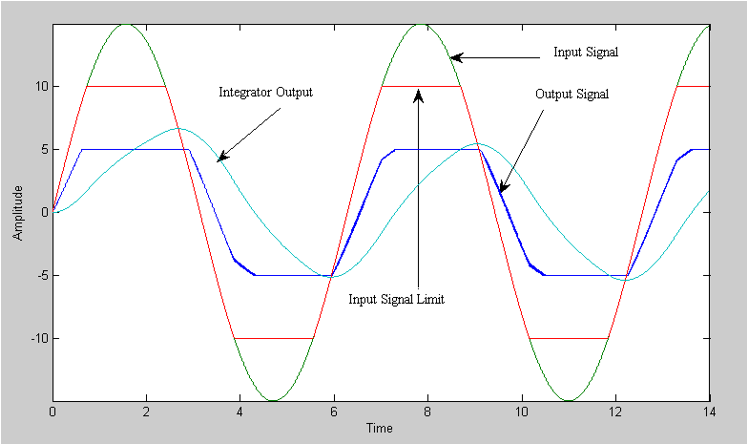Figure 2: Showing different signals from the SIMULINK model shown in fig. 1.

## Step 2: Software Configurations

In this section; the objectives are:
I) Development of the SIMULINK model for regular PID, PID with anti-windup compensation and actuator saturation compensation separately.
II) A SIMULINK model which considers all the above three scenarios at the same time.

Objective I

Regular PID Controller
There are three different forms of PID controllers .
• The standard or non-interacting form
• The series or interacting form
• The parallel formFigure 3: SIMULINK model of the regular PID for controlling the plant.Figure 4: Showing different signals from the SIMULINK model shown in fig. 3.

In this study, the standard form of PID controller is implemented and the SIMULINK model is shown in figure 3. The PID gains are kp=2, ki=1, kd=0.4. The signal in the signal generator is selected as square wave and the frequency and amplitude are set to 0.2 cycle/second and 3 units respectively. The saturation limit is set to ±5V. In real life, the derivative controller is cascaded with a first order low pass filter as to avoid unexpected noise amplification  which is depicted in figure 3. So, the structure of the PID controller becomes

Where, u(s) is the control signal and e(s) is the error signal. The proportional gain, integral and derivative time are denoted by Kp, Ti and Td respectively. N is the selection number which has significant effects on the controller performance for its different values. In this case, N is set to 20.
The plant model (DC motor) is simulated according to the instruction which is given by following transfer function.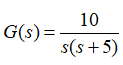Figure 4 shows the different signals from the system. The color ‘green’ is the input signal, ‘blue’ is the output signal from the plant, ‘red’ is the PID controller signal and ‘light blue’ is the output from the integrator. These represent ordinary performance of the controller. The controller performance is 30.09 and integrated absolute error is 34.71 which resemble ordinary performance of the controller based on the lab manual parameter values. This could eventually be improved by taking some actions.

PID controller with anti-windup compensation
In this case, integrator windup is taken under consideration and an anti-windup compensator is designed according to the lab instruction. Following figure 5 shows the SIMULINK model of the system where anti-windup compensation is taken into account. The anti-windup feedback gain is set to 0.5. The rest of the parameters are same as the regular PID controller. Figure 6 highlights the effect of anti-windup compensation on the signals from plant, controller and integrator. These results show some improvements from the previous case. Figure 7 shows the controller performance and integrated absolute error which are 25.97 and 28.78 respectively. Although it has been stated that the performance is increased in this case, but the phenomenon “Integrator Windup” is still in the system.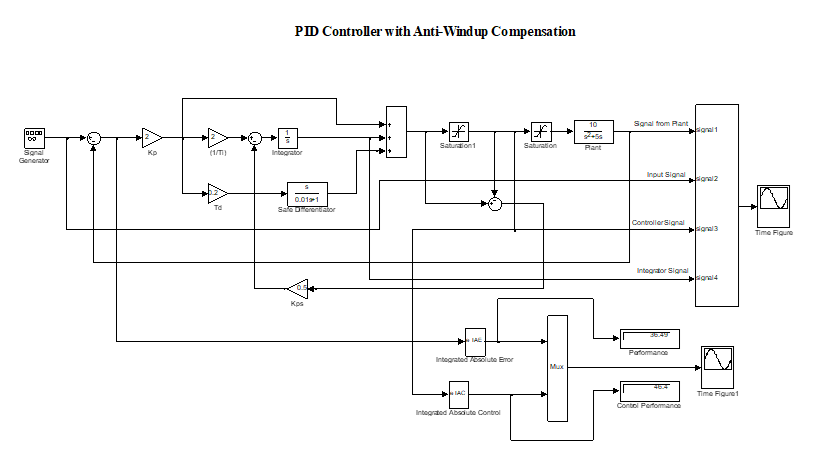Figure 5: SIMULINK model of the PID controller having anti-windup compensation.Figure 6: Showing different signals from the SIMULINK model shown in fig. 5.Figure 7: Showing IAE and IAC for the PID controller with anti-windup compensation.

Saturation Conditioned Controller
This is the last category where actuator saturation using Hanus conditioning method is explored. For this case, the controller may be implemented by following equations based on Hanus conditioning for actuator saturation.

(2)

(3)

Here, v is the actual control signal which the plant will experience. The controller is then formed by state space model which is seen in figure 8. The parameters are kept same in the SIMULINK model for convenience and comparison. The A, B, C and D matrices of the controller are evaluated from equations (2) and (3). Figure 9 represents input signal (light blue), control signal (red), saturated control signal (green) and plant response (blue). The controller performance and integrated absolute error are 33.26 and 26.58 respectively which is shown by figure 10.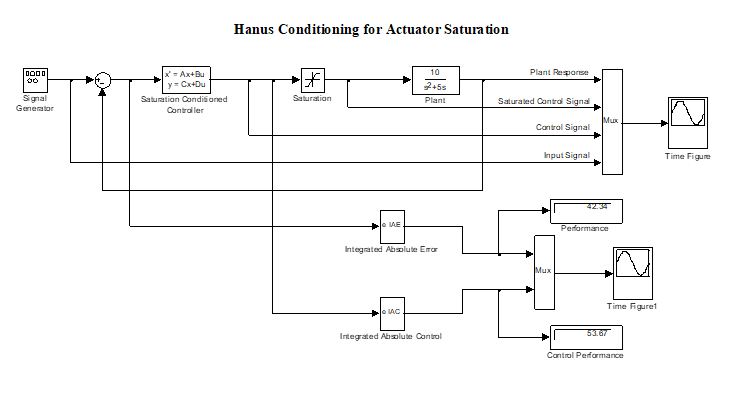Figure 8: SIMULINK model of the saturation conditioned controller implemented to control the plant.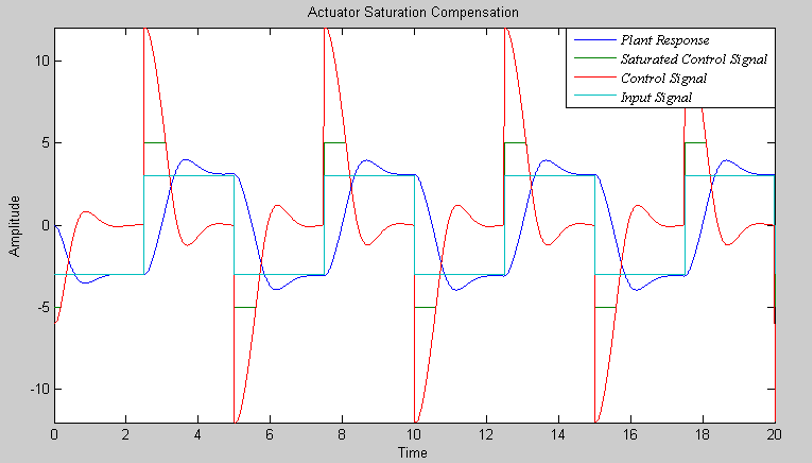Figure 9: Showing different signals from the SIMULINK model shown in fig. 8.Figure 10: Showing IAE and IAC for the actuator saturation control.

Objective II

A Combined Model Considering PID, PID with Anti-Windup and Actuator Saturation Compensation

Figure 11 represents the SIMULINK model which considers all the three controllers in a single model. Three subsystems are generated for forming three different controllers: regular PID, PID having anti windup compensation and actuator saturation compensation. The manual switches in the model connect the three controllers individually which basically depend on the requirement of the system.Figure 11: A combined SIMULINK model considers regular PID, PID with anti-windup compensation and actuator saturation compensation.

PID with anti-windup compensator has the ability to consider the following three controller models as per the instruction.
·         Anti-windup compensation with local feedback
·         Integrator input is set to zero when the control signal is saturated.
·         Local positive feedback of a first order system around a software saturation block

Figure 12 shows the desired PID with anti-windup compensator model. It is worth to be highlighted here separately as to emphasize the modeling steps of three controllers which compensate integrator windup.
The configuration of the anti-windup compensation via local feedback was developed earlier. The second configuration (zero integrator input while actuator saturates) is designed simply by adding a manual switch before the integrator. When the possibility of actuator saturation is observed, the switch can be turned off to make integrator contribution to the controller zero. The last configuration which is the addition of a first order system as positive feedback around a saturation block is modeled which is shown in figure 12. Here, a lag compensator is considered as controller which is obtained from the previous Hanus state-space model by simply converting to a transfer function. The following MATLAB commands show this step:

>> A=-0.5;
>> B=0.5;
>> C=1;
>> D=2;
>> [num, den] = ss2tf (A,B,C,D)
num =
2.0000    1.5000
den =
1.0000    0.5000
>> tf ( num, den )

Transfer function:
2 s + 1.5
---------
s + 0.5

Figures 13, 14 and 15 show the results of the three control setups: anti-windup compensation via local feedback, zero integrator input while actuator saturates and addition of a first order system as positive feedback around a saturation block all in the PID controller with anti-windup compensation controller respectively.
Regarding performance evaluation, controller with anti-windup compensation by local feedback shows better results than other two controllers. The Integrated Absolute Error (IAE) and Integrated Absolute Control (IAC) are 28.78 and 25.97 respectively. The Integrated Absolute Error (IAE) and Integrated Absolute Control (IAC) of the controller which does the integrator input zero while actuator saturates are 34.71 and 61.37 respectively. And, finally Integrated Absolute Error (IAE) and Integrated Absolute Control (IAC) of the controller which adds a first order system as positive feedback around a saturation block are 31.98 and 38.78 respectively. So, from these comparisons, the second type of the controller configuration gives the worst performance among the three controllers.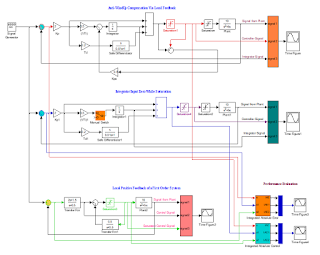Figure 12: A SIMULINK model shows PID with anti-windup compensation having three alternative controller models according to the lab instructions.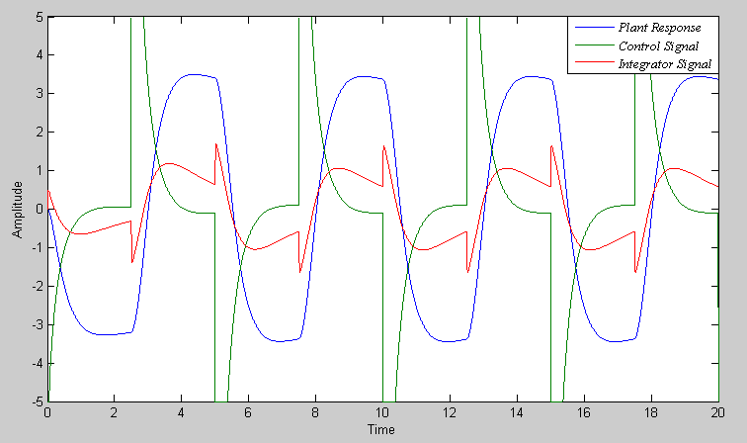Figure 13: Showing responses when anti-windup compensation via local feedback of the difference between the saturated and unsaturated control signal is considered.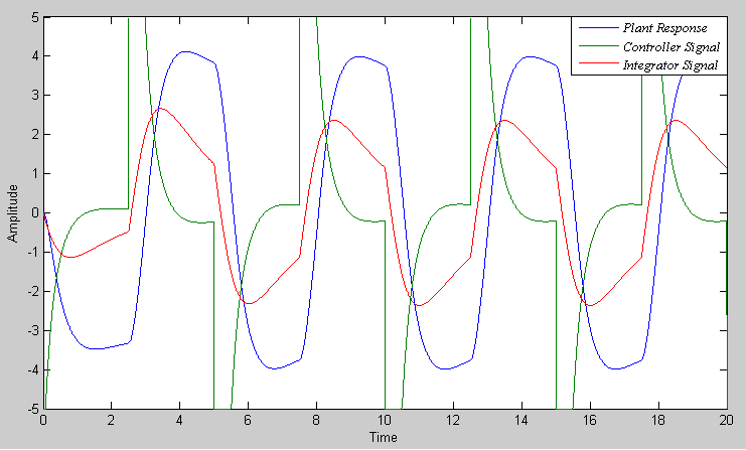Figure 14: Showing output signals of the model where the integrator input is set to zero when the control signal gets saturated.Figure 15: Showing output signals of the model when local positive feedback of a first order system around a software saturation block is implemented.

## Step 3: Experimental Testing

In the previous sections, rigorous analysis on simulation results give good understanding of the ‘wind-up’ phenomenon and some controllers performance to skip that situation. In this section, experiment is carried out to further realize the ‘wind-up effects’ in depth and comparison between simulation and experiment results. For the experiment, a SIMULINK model is designed and modified further based on the original “SIMULINK Model of DC Motor Control” of the EEEL-155 lab which is shown in figure 16. Figure 17 shows the model of the three controller configurations: PID, PIDAW, and ASC. Manual switches are inserted to have the provision for the controller selection. The controllers’ parameters are kept same in the experiment to enlighten a fair comparison. Figures 18, 19, and 20 show the physical DC motor responses for each of the three controllers (PID, PIDAW, and ASC) implementation respectively. By observing these figures, ASC controller shows better performance than the other two controllers. Although in all the three cases, ‘wind-up’ phenomenon is still present, nevertheless the ASC controller (figure 20) implementation decreases the DC motor transients more than the other two controllers. It should be mentioned here, keeping the controllers’ parameters same as the lab manual instruction does not prevent the ‘wind-up’ effect completely which could be done by further tuning the controller parameters. At the end of this report, a table is provided which shows the comparison between the simulation and experiment results.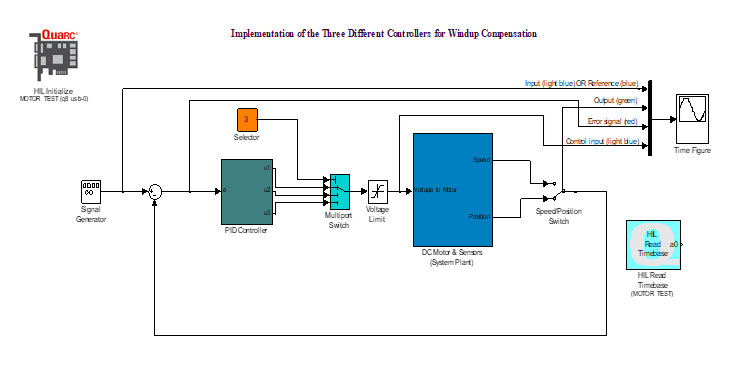Figure 16: A combined SIMULINK model linked with physical DC motor for experimental validation of the controllers developed in objective II.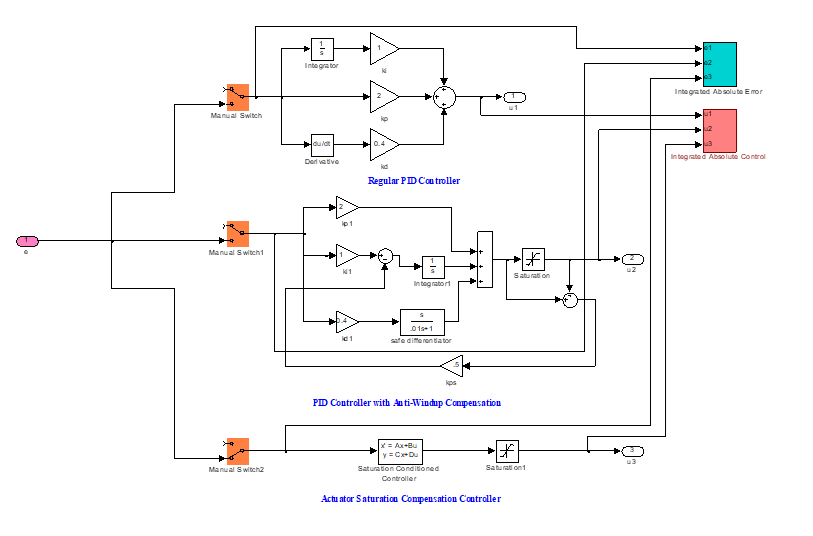Figure 17: Controller model considers regular PID, PID with anti-windup compensation and actuator saturation compensation.Figure 18: Showing the action of regular PID controller on the experimental DC motor.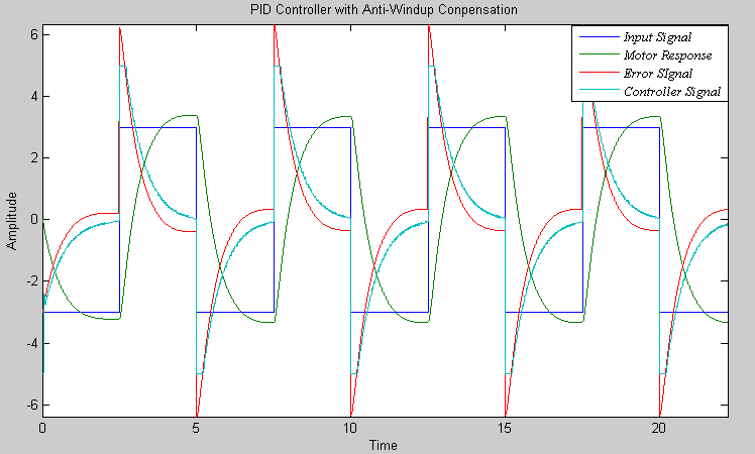Figure 19: Showing the action of PID controller with anti-windup compensation on the experimental DC motor.Figure 20: Showing the action of saturation compensation on the experimental DC motor.

Following table 1 gives the comparison of the results for implementing different controller configurations between simulation and experiment. This comparison is considered as qualitative as the actual DC motor is certainly non-linear whereas in simulation a simple transfer function is considered according to the lab manual. However, this comparison does not show huge deviation of the simulation results from the experiment. Moreover, both the simulation and experimental results are close comparing the three controllers’ performance indices.
In conclusion, this lab work gives the scope to observe and dig into the one special non-linear phenomenon of the system when there is the ‘wind-up’ and further furnishes the ability to design anti-windup compensators to avoid this consequence.

Table 1: Comparison between Simulation and Experiment Results

 Controller Types Performance Index Simulation Results Experimental Results Regular PID IAE 34.71 28.25 IAC 30.09 32.16 PID with Anti-Windup Compensation (PIDAW) IAE 28.78 25.51 IAC 25.97 29.51 Actuator Saturation Compensation (ASC) IAE 26.58 20.26 IAC 33.26 28.29

References

 Advanced PID control / Karl Johan Astrom and Tore Hagglund, ISA - Instrumentation, Systems, and Automation Society, 2006.

 Dingyü Xue, YangQuan Chen and Derek P. Atherton, Linear Feedback Control: Analysis and Design with MATLAB, the Society for Industrial and Applied Mathematics, 2007.